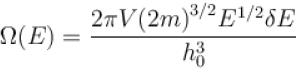Courses

# Statistical Physics MCQ Level – 2

## 10 Questions MCQ Test Topic wise Tests for IIT JAM Physics | Statistical Physics MCQ Level – 2

Description
This mock test of Statistical Physics MCQ Level – 2 for IIT JAM helps you for every IIT JAM entrance exam. This contains 10 Multiple Choice Questions for IIT JAM Statistical Physics MCQ Level – 2 (mcq) to study with solutions a complete question bank. The solved questions answers in this Statistical Physics MCQ Level – 2 quiz give you a good mix of easy questions and tough questions. IIT JAM students definitely take this Statistical Physics MCQ Level – 2 exercise for a better result in the exam. You can find other Statistical Physics MCQ Level – 2 extra questions, long questions & short questions for IIT JAM on EduRev as well by searching above.
QUESTION: 1

### If Z be the partition function and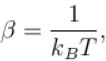then the average energy of the system is given by. Select one:

Solution:

Average energy of a system is defined as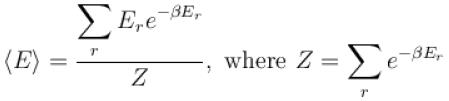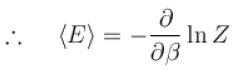The correct answer is: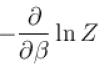QUESTION: 2

### For a Fermi gas of N particles in three dimension at T = 0K, the Fermi energy Ef is proportional to Select one:

Solution:

The Fermi energy is given by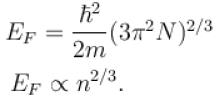QUESTION: 3

### Gibb’s paradox arises due to. Select one:

Solution:

In a semi-classical derivation of entropy that does not take into account the indistinguishability of particles, yields an expression for the entropy which is not extensive. This is called Gibb’s Paradox.
The correct answer is: Distinguishability of classical particles

QUESTION: 4

Which of the following relations between the particle number density n and temperature T must hold good for a gas consisting of non-interacting particles to be described by quantum statistics?
Select one:

Solution:

The particle density and temperature T are related as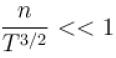The correct answer is: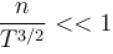QUESTION: 5

When an ideal quantum gas can be treated as an ideal classical gas?
Select one:

Solution:

Let us take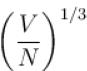where V is volume and N is number of particles.
Now, for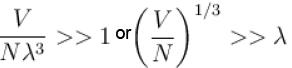i.e. when interparticle is much larger than the thermal de-Broglie wavelength, the gas will obey Maxwell Boltzmann Statistics.
The correct answer is: When de-Broglie wavelength is much smaller than the inter-particle distance

QUESTION: 6

What is the partition function of a monoatomic gas contained in value V, at temperature T and pressure p
Select one:

Solution: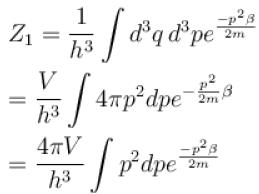Let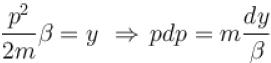∴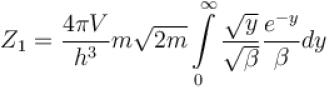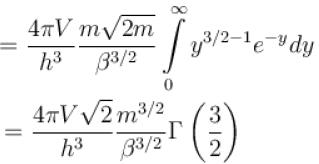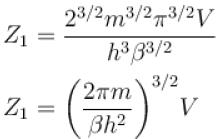∴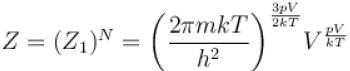The correct answer is: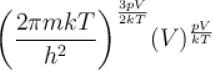QUESTION: 7

Consider a one level system having energy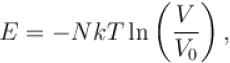where V0 is a constant and symbols have usual meanings, the partition function for the system is.
Select one:

Solution:

Partition function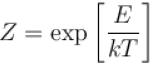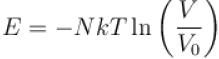⇒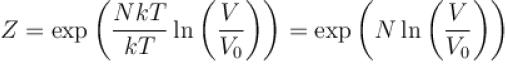The correct answer is: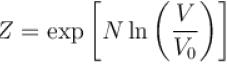QUESTION: 8

The partition function of a single gas molecule is Zα. The partition function of N such non-interacting gas molecules is then given by
Select one:

Solution:

Partition function of single gas molecule = Zα
Since N particles are non-interacting, the partition function =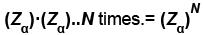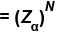The correct answer is: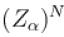QUESTION: 9

The classical partition function Z gives the
Select one:

Solution:

Classical partition function Z gives nothing but sum of all the possible states. In discrete systems, it gives basically the sum over all the microstates and in continuous systems it is represented in terms of integrating.
The correct answer is: Sum of states of the system

QUESTION: 10

For a single particle of mass m enclosed in a volume V. the number of accessible microstate in energy range E to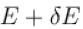is given by the (the phase space is divided by the rule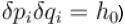Select one:

Solution:

The phase space volume is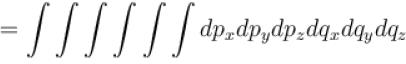where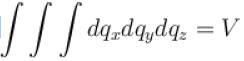and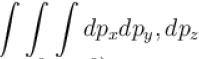is limited by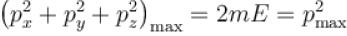Hence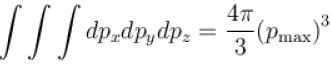∴ Volume of phase space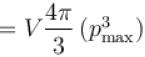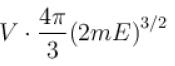Number of phase space =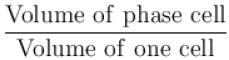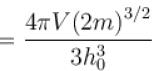The number of microstates in the energy range E to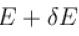is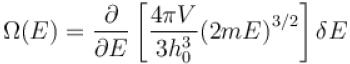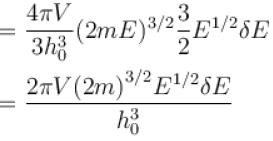The correct answer is: